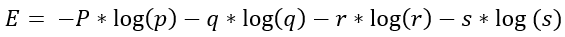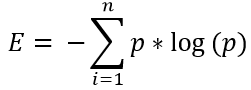Classification

Decision Tree

Machine Learning

Regression

January 8, 2021

# What is Entropy? How is it calculated?

Entropy is the measure of randomness in data. Randomness signifies the heterogeneity of labels. Decision trees split the data in manner that leads to decrease in entropy. Thus Decision Trees aim to divide the data with heterogenous labels into subsets/sub-regions of data with homogenous labels. Thus with each division level of homogeneity increases and entropy decreases. In fact entropy is the cost function that decision trees employ as basis of splitting the data, if the the split leads to decrease in entropy then it’s carried out else not.

For a dataset with 4 labels- say a, b, c, d – with probability of occurrence of each label being p, q, r, s respectively, then the entropy of the data would be given by the following equation:For a dataset with n classes, the formula would be:Where p is the probability of occurrence of each class.

by : Monis Khan

Quick Summary:

Entropy is the measure of randomness in data. Randomness signifies the heterogeneity of labels. Decision trees split the data in manner that leads to decrease in entropy. Thus Decision Trees aim to divide the data with heterogenous labels into subsets/sub-regions of data with homogenous labels. Thus with each division level of homogeneity increases and entropy […]